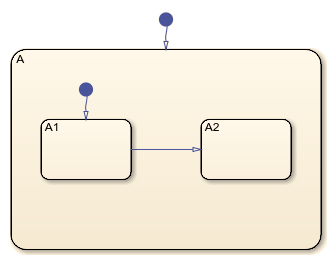# getChildren

Identify children of object

## Syntax

``objArray = getChildren(parent)``

## Description

example

````objArray = getChildren(parent)` returns an array of objects that have the specified parent.```

## Examples

collapse all

This chart has two children, state `A` and a default transition. State `A` has four children, state `A1`, state `A2`, and two transitions.Open the model and access the `Stateflow.Chart` object for the chart.

```open_system("sfHierarchyAPIExample") ch = find(sfroot,"-isa","Stateflow.Chart");```

Identify the children of the chart. Display the object types of the children.

```children = getChildren(ch); classes = arrayfun(@class,children,UniformOutput=false)```
```classes = 2x1 cell {'Stateflow.State' } {'Stateflow.Transition'} ```

One element in `children` is a state. Display the name of the state.

```idx = (classes=="Stateflow.State"); state = children(idx); state.Name```
```ans = 'A' ```

Identify the children of state `A`. Display the object types of the children.

```grandchildren = getChildren(state); classes = arrayfun(@class,grandchildren,UniformOutput=false)```
```classes = 4x1 cell {'Stateflow.State' } {'Stateflow.State' } {'Stateflow.Transition'} {'Stateflow.Transition'} ```

Two elements in `grandchildren` are states. Display the names of the states.

```idx = (classes=="Stateflow.State"); grandchildren(idx).Name```
```ans = 'A1' ```
```ans = 'A2' ```

collapse all

## Version History

Introduced before R2006a